CBSE Class 10 Sample Paper for 2021 Boards - Maths Standard

Class 10
Solutions of Sample Papers for Class 10 Boards

## If tan⁡A=3/4 , find the value of 1/sin⁡A +1/cos⁡A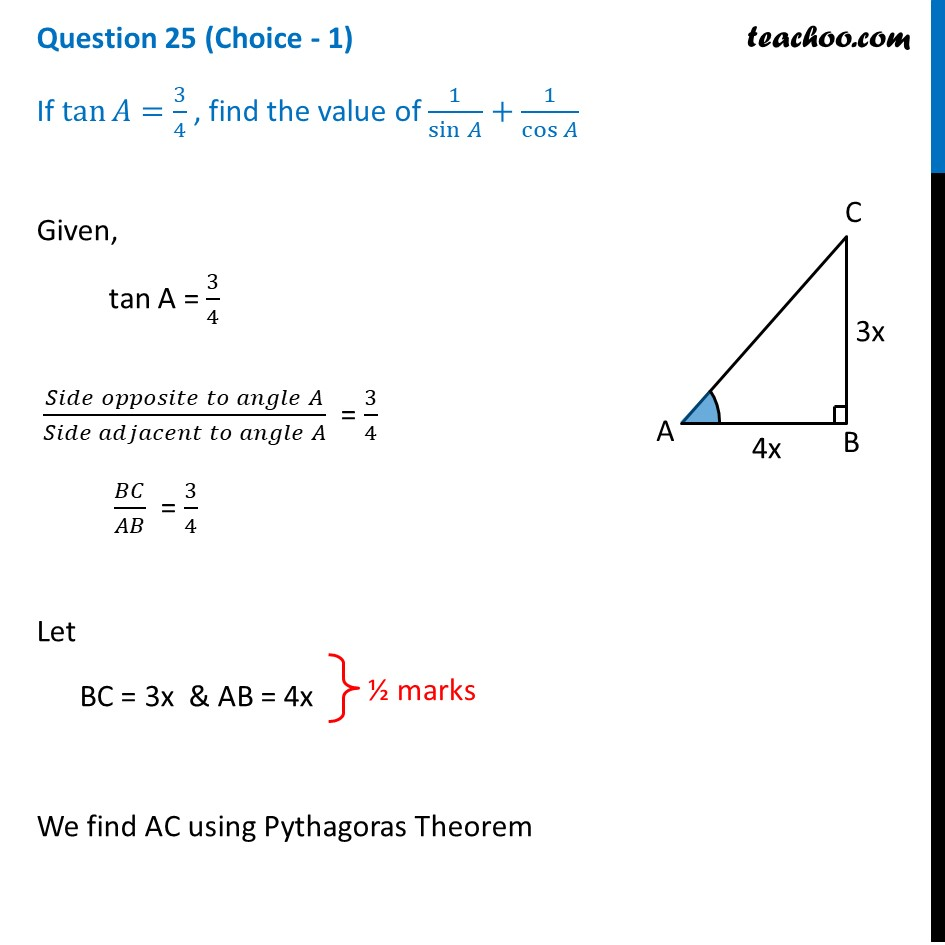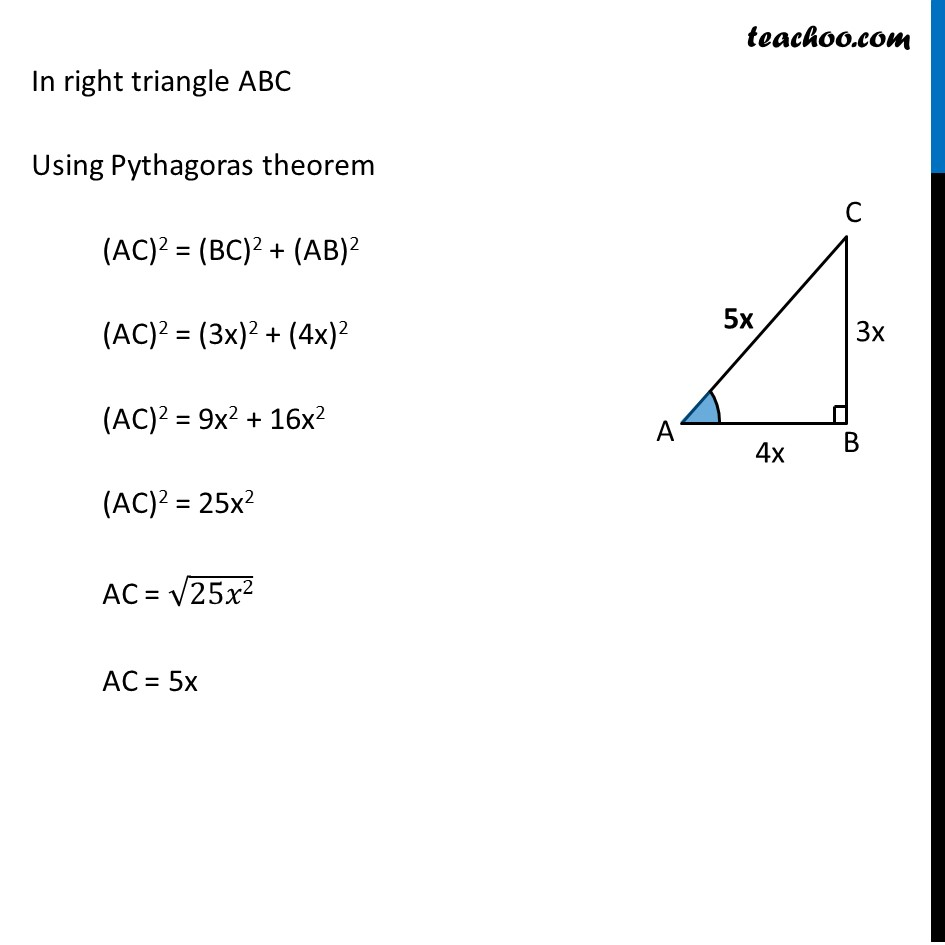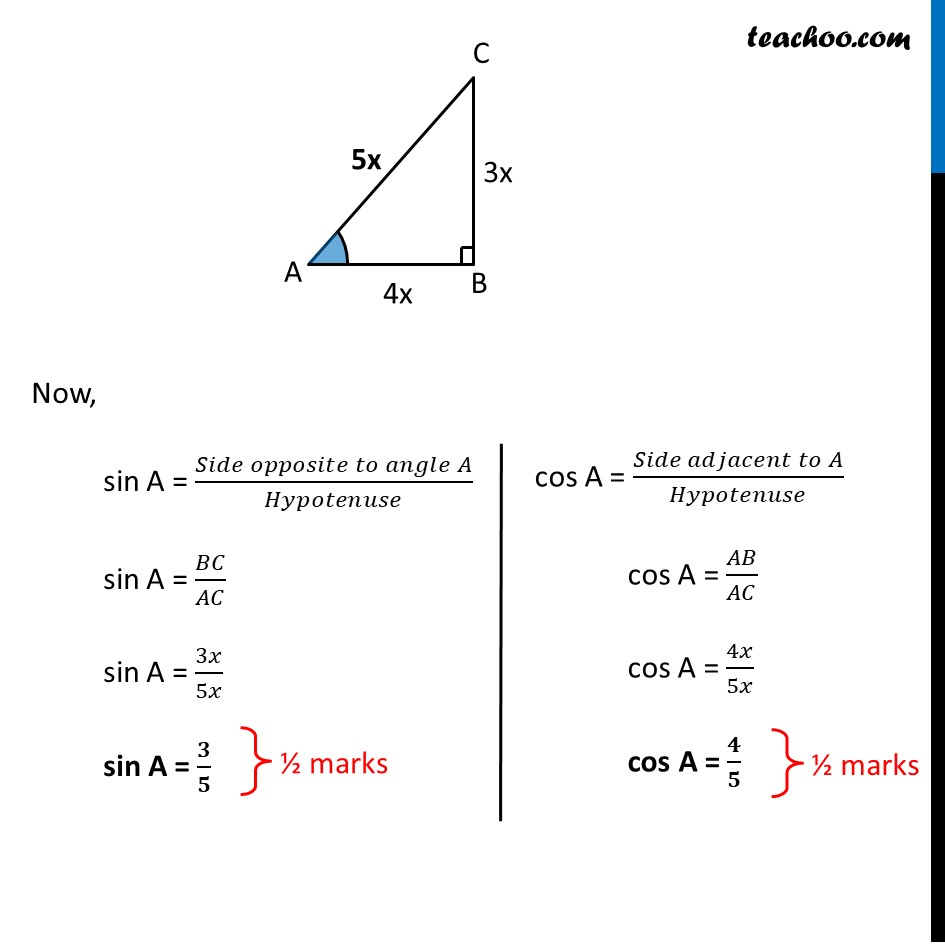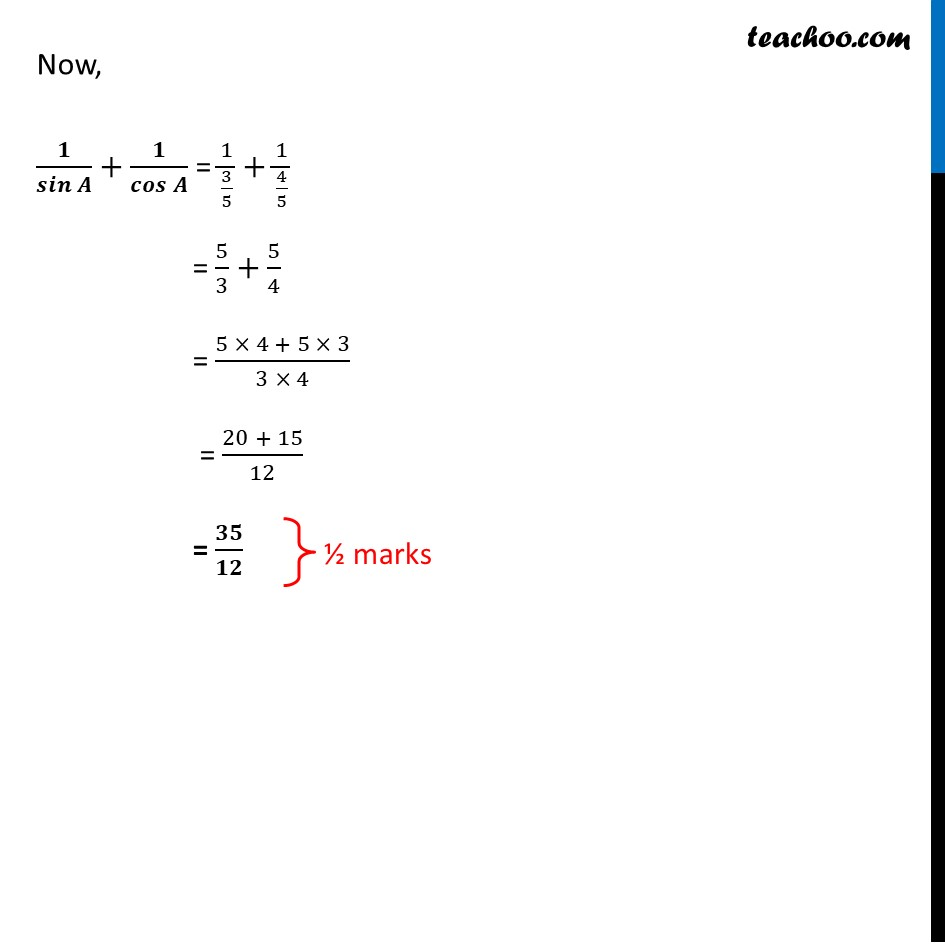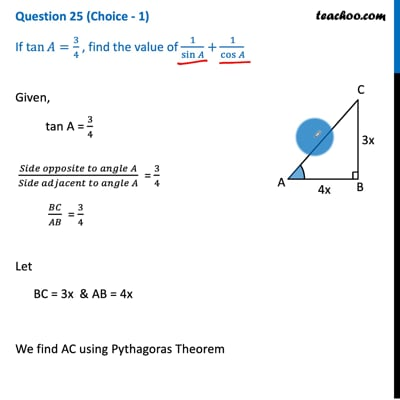This video is only available for Teachoo black users

Introducing your new favourite teacher - Teachoo Black, at only ₹83 per month

### Transcript

Question 25 (Choice - 1) If tan⁡𝐴=3/4 , find the value of 1/sin⁡𝐴 +1/cos⁡𝐴 Given, tan A = 3/4 (𝑆𝑖𝑑𝑒 𝑜𝑝𝑝𝑜𝑠𝑖𝑡𝑒 𝑡𝑜 𝑎𝑛𝑔𝑙𝑒 𝐴)/(𝑆𝑖𝑑𝑒 𝑎𝑑𝑗𝑎𝑐𝑒𝑛𝑡 𝑡𝑜 𝑎𝑛𝑔𝑙𝑒 𝐴) = 3/4 𝐵𝐶/𝐴𝐵 = 3/4 Let BC = 3x & AB = 4x We find AC using Pythagoras Theorem In right triangle ABC Using Pythagoras theorem (AC)2 = (BC)2 + (AB)2 (AC)2 = (3x)2 + (4x)2 (AC)2 = 9x2 + 16x2 (AC)2 = 25x2 AC = √25𝑥2 AC = 5x Now, sin A = (𝑆𝑖𝑑𝑒 𝑜𝑝𝑝𝑜𝑠𝑖𝑡𝑒 𝑡𝑜 𝑎𝑛𝑔𝑙𝑒 𝐴)/𝐻𝑦𝑝𝑜𝑡𝑒𝑛𝑢𝑠𝑒 sin A = 𝐵𝐶/𝐴𝐶 sin A = 3𝑥/5𝑥 sin A = 𝟑/𝟓 cos A = (𝑆𝑖𝑑𝑒 𝑎𝑑𝑗𝑎𝑐𝑒𝑛𝑡 𝑡𝑜 𝐴)/𝐻𝑦𝑝𝑜𝑡𝑒𝑛𝑢𝑠𝑒 cos A = 𝐴𝐵/𝐴𝐶 cos A = 4𝑥/5𝑥 cos A = 𝟒/𝟓 Now, 𝟏/𝒔𝒊𝒏⁡𝑨 +𝟏/𝒄𝒐𝒔⁡𝑨 = 1/(3/5)+1/(4/5) = 5/3+5/4 = (5 × 4 + 5 × 3)/(3 × 4) = (20 + 15)/12 = 𝟑𝟓/𝟏𝟐